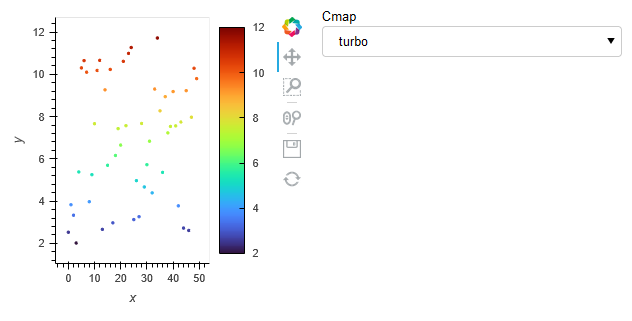# Rescale / transform y axis of DynamicMap scatter

I have an app with streaming data displayed in a scatter plot using `hv.DynamicMap`. The y data of the scatter is in units of J/mol, but I’d like the users to interact with the data in units of kJ/mol.

Currently I’m rescaling the data with a factor 1e3 before plotting it. The data is plotted with a colormap so I’m also rescaling the `clim` opt.
However, in other parts of the app I’m also using the same data and colormap and as a consequence the step of rescaling appears in many parts of my app.

I’m trying to rescale/transform the data just before plotting such as described in this hvplot issue here.

How can I do the equivalent thing in HoloViews? I’ve been playing with `hv.dim` but I seem to be unable to get that to work.

Also, it it possible to somehow pass the `norm` object to opts directly instead of `clim` ?

MWE of what I’m currently doing with rescaling:

``````
import holoviews as hv
import numpy as np
import pandas as pd
import panel as pn
from functools import partial
import param
from holoviews.streams import Pipe
from matplotlib.colors import Normalize

hv.extension('bokeh')

class Controls(param.Parameterized):
cmap = param.Selector(default='turbo', objects=['turbo', 'inferno', 'viridis'])

x = np.arange(50)
y = 10000*(np.random.rand(50)+0.2)
sclf = 1e-3

df = pd.DataFrame({'x': x, 'y': y*sclf})

ymin, ymax = 2000, 12000
norm = Normalize(vmin=ymin, vmax=ymax)
clim = (norm.vmin*sclf, norm.vmax*sclf)

stream = Pipe(data=df)
controls = Controls()

func = partial(hv.Scatter, kdims=[None, 'y'])
plot = hv.DynamicMap(hv.Scatter, streams=[stream])
transform = {'y': hv.dim('y')*1e-3}
s = plot.apply.opts(cmap=controls.param['cmap'], color='y', clim=clim, colorbar=True)

hv_pane = pn.pane.HoloViews(s)
app = pn.Row(hv_pane, pn.panel(controls.param.cmap))

app.servable()

``````

App:I’m not sure I’m following all the details here, but a DynamicMap can do any arbitrary function, so if you’re already using DynamicMap, seems like you can fold any rescaling you want into that:

``````import holoviews as hv
import numpy as np
import pandas as pd
import param
from holoviews.streams import Pipe
from matplotlib.colors import Normalize

hv.extension('bokeh')

x = np.arange(50)
y = 10000*(np.random.rand(50)+0.2)
sclf = 1e-3

df = pd.DataFrame({'x': x, 'y': y})

ymin, ymax = 2000, 12000
norm = Normalize(vmin=ymin, vmax=ymax)
clim = (norm.vmin*sclf, norm.vmax*sclf)

stream = Pipe(data=df)

def scaled_scatter(data):
d = data.copy()
d.y *= sclf
return hv.Scatter(d)

plot = hv.DynamicMap(scaled_scatter, streams=[stream])
plot.apply.opts(cmap='turbo', color='y', clim=clim, colorbar=True)
``````

Thanks, I’ve updated my code and I’m rescaling the data before sending it to the plot as you suggest.

I guess my question wasn’t too clear but I’ll try to rephrase. I was expecting the following to work:

``````ydim = hv.dim('y')*1e-3
plot = hv.Scatter(df, kdims=['x'], vdims=[ydim])
s = plot.apply.opts(cmap=controls.param['cmap'], color=ydim, clim=clim, colorbar=True)
``````

This does not work as a `hv.dim` is not a valid type for `vdims`, it must be `tuple`, `str`, `dict` or `Dimension`. However I can pass the `hv.dim` to the `color` keyword argument of `opts` and does rescale the data before applying the colors.

My question: is there some other construction I can use which I can pass to both `vdims` and `color` to rescale both the ydata and the color data?